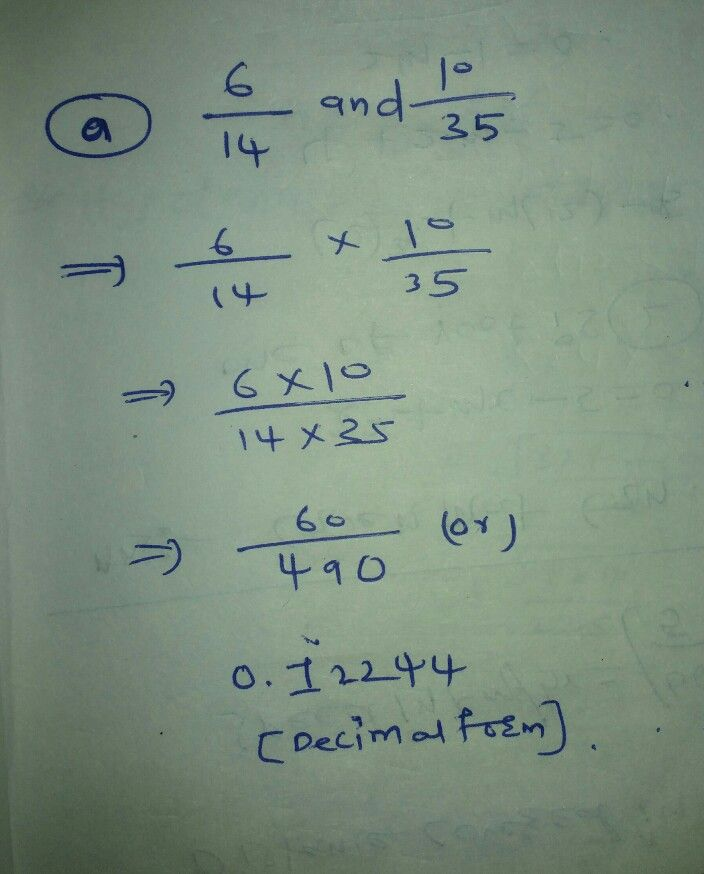Symbol
ProblemWhich of the following pairs of rational numbers are equvalent? a. $\dfrac {6} {14}$ and $\dfrac {10} {35}$ b. $\dfrac {6} {22}$ and $\dfrac {-22} {6}$ C. $\dfrac {-7} {35}$ and $\dfrac {2} {-10}$ d. $\dfrac {-8} {10}$ and $\dfrac {-20} {25}$
7th-9th grade
Other
SolutionQanda teacher - sweetieplease waitStudent
thanks
?Qanda teacher - sweetie
give me rating